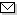Language:   Search:   Contact# Search and Browse the MSC 2000

Search:
Please note that in the second half of 2009, MSC2010 is deployed in the production of ZMATH and will become official standard in January 2010.

Enter a query and click »Search«...

35-XX Partial differential equations → ZMATH
35-00 General reference works (handbooks, dictionaries, bibliographies, etc.) → ZMATH
35-01 Instructional exposition (textbooks, tutorial papers, etc.) → ZMATH
35-02 Research exposition (monographs, survey articles) → ZMATH
35-03 Historical (must also be assigned at least one classification number from Section 01) → ZMATH
35-04 Explicit machine computation and programs (not the theory of computation or programming) → ZMATH
35-06 Proceedings, conferences, collections, etc. → ZMATH
35Axx General theory → ZMATH
35Bxx Qualitative properties of solutions → ZMATH
35Cxx Representations of solutions → ZMATH
35Dxx Generalized solutions of partial differential equations → ZMATH
35Exx Equations and systems with constant coefficients [See also 35N05] → ZMATH
35Fxx General first-order equations and systems → ZMATH
35Gxx General higher-order equations and systems → ZMATH
35Hxx Close-to-elliptic equations → ZMATH
35Jxx Partial differential equations of elliptic type [See also 58Jxx, 58J10, 58J20] → ZMATH
35Kxx Parabolic equations and systems [See also 35Bxx, 35Dxx, 35R30, 35R35, 58J35] → ZMATH
35Lxx Partial differential equations of hyperbolic type [See also 58J45] → ZMATH
35Mxx Partial differential equations of special type (mixed, composite, etc.) {For degenerate types, see 35J70, 35K65, 35L80} → ZMATHAbel prize 2010I. M. Gelfand 1913-2009MSC2010